# Volume + velocity - math problems

#### Number of problems found: 15

• Velocity ratio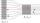Determine the ratio at which the fluid velocity in different parts of the pipeline (one part has a diameter of 5 cm and the other has a diameter of 3 cm), when you know that at every point of the liquid is the product of the area of tube [S] and the fluid
• Water flowHow much water flow in pipe with a diameter of 16 cm in 1 hour if the water velocity is 2.5 m/s?
• PumpsPump that draws water at velocity 3.5 liters per second water from a construction trench take 35 minutes. a) Find out how many minutes the water would run out of the trench pump that draws 7.4 liters of water per second. b) What is the pumping velocity wo
• A filterIt is a pool with a volume of 3500 liters. The filter filters at 4m cubic per hour. How many minutes would it filter the entire pool?
• Trough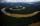How many liters of water per second can go via trough, which has a cross section of semicircle with radius 2.5 m and speed of water is 147 cm per second?
• Water flow 2How many litres of water will flow in 7 minutes from a cylindrical pipe 1 cm in diameter, if the water flows at a speed of 30 km per hour
• Fuel mileage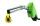You have 3/8 tank of fuel. Estimate fuel consumption @ 6mpg. Estimate speed @ 50mph. Your fuel tank holds 200 gals. Figure out fuel mileage.
• Water tankThe water tank has a cylindrical shape with a base diameter of 4.2 m and is 80 cm deep. How many minutes will take fill it 10 cm below the edge of the tank if water flowing 2 liters per second?
• Water level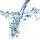To cuoid shaped poll, bottom size 2m and 3.5m; flows water at a rate of 50 liters per minute. How long will take to water reach level 50 cm?
• Two water containers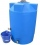In the first container, there are 200 m3 of water and in the second 40 m3. The first container will flow down at a rate of 10 m3 water per hour. At the same time flows to the second rate of 5 m3 per hour. After how many hours there will be three times les
• Workers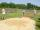Workers digging a jump pit in the school yard. Pit has a cuboid shape with a length 12 m, a width 20 dm and depth 36 cm. They excavate 0.4 cubic meters of soil an hour. How much time (hours and minutes) is need to the excavate this pit?
• PipesThe water pipe has a cross-section 1087 cm2. An hour has passed 960 m3 of water. How much water flows through the pipe with cross-section 300 cm2 per 9 hours if water flows the same speed?
• Water channelThe cross section of the water channel is a trapezoid. The width of the bottom is 19.7 m, the water surface width is 28.5 m, the side walls have a slope of 67°30' and 61°15'. Calculate how much water flows through the channel in 5 minutes if the water flo
• Two cylinders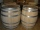Two cylinders are there one with oil and one with an empty oil cylinder has no fixed value assume infinitely. We are pumping out the oil into an empty cylinder having radius =1 cm height=3 cm rate of pumping oil is 9 cubic centimeters per sec and we are p
• Orlík hydroelectric plantThe Orlík hydroelectric power plant, built in 1954-1961, consists of four Kaplan turbines. For each of them, the water with a flow rate of Q = 150 m3/s is supplied with a flow rate of h = 70.5 m at full power. a) What is the total installed power of the p

Do you have an interesting mathematical word problem that you can't solve it? Submit a math problem, and we can try to solve it.

We will send a solution to your e-mail address. Solved examples are also published here. Please enter the e-mail correctly and check whether you don't have a full mailbox.

Please do not submit problems from current active competitions such as Mathematical Olympiad, correspondence seminars etc...

Tip: Our volume units converter will help you with the conversion of volume units. Do you want to convert velocity (speed) units? Volume - math word problems. Velocity - math word problems.